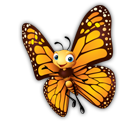Program OutlineThis comprehensive program provides thorough coverage of Math skills at a K-1 level.

## Numeration

### Unit 1: Learning the Numbers

This Level K-1 unit teaches the student to identify numbers by sight, sound and counting. It introduces the abacus as a counting tool and how to add multiple digits.
44 Lessons

### Unit 2: Working with Numbers

Level K-1 introduces counting up by 1s, 5s and 10s. Students learn to count backwards by 1s. Comparing numbers to determine which are greater and smaller is emphasized along with ordering numbers.
45 Lessons

This Level K-1 unit focuses on the understanding of two digit addition. It emphasizes how problem solving can be used in row and column addition practices.
19 Lessons

### Unit 4: Subtraction

This Level K-1 unit works on understanding two digit subtraction through removing items from a group, using the basic row concept and the identification of the correct answer. It teaches students the difference between addition and subtraction and how they are opposites.
15 Lessons

### Unit 5: Calculator Practice

This Level K-1 unit introduces the basic understanding of single digit addition and subtraction using a calculator.
4 Lessons

### Unit 6: Money

This Level K-1 unit introduces currency and the values and names of different coins. Relating back to addition, it teaches the students how to add coins together to end up with a sum of money.
37 Lessons

### Unit 7: Mixed Practice

The focus of this Level K-1 unit is further understanding addition and subtraction for 2 digit problems. It introduces the concept of equality using an equal sign in an equation.
8 Lessons

## Patterning

### Unit 1: Attributes

This Level K-1 unit works on identifying similar characteristics of objects such as shape and color. Sorting like and different objects is used to practice the concept.
8 Lessons

### Unit 2: What Comes Next?

Level K-1 students learn to determine patterns using pictures, letters and numbers. They learn to predict what comes next in a sequence.
6 Lessons

### Unit 3: What is Missing?

Level K-1 students learn to determine patterns to complete the sequence using pictures, letters and numbers.
7 Lessons

### Unit 4: Make a Pattern

Further understanding in Level K-1 of patterns is emphasized by continuing a pictorial pattern by choosing the correct set of items.
4 Lessons

### Unit 5: Talking About Patterns

Further focus in Level K-1 on determining basic arithmetic and geometric patterns through recognition of shapes and numbers. The student will learn to recognize if the pattern is increasing or decreasing.
4 Lessons

### Unit 6: Number Charts

This Level K-1 unit teaches students how to use a number chart with arithmetic patterns that increase and decrease by 1.
3 Lessons

## Measurement

### Unit 1: Days, Months, Seasons

This Level K-1 unit introduces the calendar, understanding the months and days of the week and their order.
27 Lessons

### Unit 2: Telling Time

This Level K-1 unit focuses on understanding the passage of time. It works with pictorial sequencing of events. The unit also teaches how to tell time to the half hour using digital and analog clocks.
11 Lessons

### Unit 3: Temperature

This Level K-1 unit is about temperature and understanding concepts like warmer, cooler, hot, cold. It relates these concepts to everyday activities and hobbies.
5 Lessons

### Unit 4: Length and Height

This Level K-1 unit emphasizes heights of objects through measurement and relative to one another. Students learn to measure length and height of objects.
7 Lessons

### Unit 5: Perimeter, Distance and Area

Level K-1 students measure perimeter, area and distance using standard and non-standard units. It teaches understanding the difference between perimeter and area.
7 Lessons

### Unit 6: Capacity, Volume and Mass

Level K-1 students learn to understand volume and capacity and relate it to everyday objects. Comparisons are made between objects and scales are introduced to weigh objects.
8 Lessons

## Geometry

### Unit 1: 2D Naming

Level K-1 students focus on recognizing and identifying 2D shapes such as circles, squares, rectangles and triangles.
8 Lessons

### Unit 2: 3D Naming

Level K-1 students focus on recognizing and identifying 3D shapes such as cubes, cones, cylinders and spheres.
10 Lessons

### Unit 3: 2D Properties

Level K-1 students look at the number of sides and comparing objects to understand 2D shape properties.
9 Lessons

### Unit 4: 3D Properties

This Level K-1 unit focuses on understanding 3D shape properties, comparing and classifying 3D shapes. It outlines basic properties of 3D figures - # of corners, sides, etc.
8 Lessons

### Unit 5: Symmetry

This Level K-1 unit introduces symmetry through identifying symmetrical objects and dividing objects symmetrically.
4 Lessons

### Unit 6: Directions

This Level K-1 unit provides maps and works through describing an object's location in relation to another.
6 Lessons

## Data Management

### Unit 1: Counting

This Level K-1 unit gives practice on sorting, counting and comparing objects.
4 Lessons

### Unit 2: Sorting

This Level K-1 unit gives practice with sorting objects using characteristics such as color and shape.
6 Lessons

### Unit 3: Surveying

This Level K-1 unit emphasizes how to survey data. Examples on the basic understanding and implementation of appropriate questions to collect data are included.
4 Lessons

### Unit 4: Graphing

Graphs are introduced in this Level K-1 unit. Reading and comparing graphs such as bar graphs and determining if they match data.
4 Lessons

## Probability

### Unit 1: Chance

Probability and chance are introduced in this Level K-1 unit, understanding occurrences of events in the real world.
4 Lessons